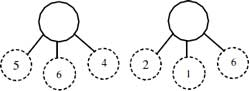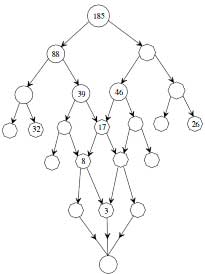# Number Bonds Worksheets

Number bond is a special concept to teach addition and subtraction. Our printable number bond worksheets for children in kindergarten through grade 3 include simple addition of two addends; identifying missing addends; adding three and four digit numbers; three addends, addition tree and number bond templates. Our free number bond worksheets are definitely worth a try!

Find the Sum:

Add the numbers and find the sum. Use the worksheets with single-digit numbers for kindergarteners and those with two-digit numbers for 1st grade and 2nd grade kids.Single-Digit: Moderate

Two-Digit: Easy

Two-Digit: Moderate

Use addition or subtraction to find the missing addend that makes the sum.Single-Digit: Moderate

Two-Digit: No Regrouping

Two-Digit: Regrouping

Number Bond: More Digits

This section is little complicating as it contains number bonds worksheet pdfs with the digits 3 and more.

Three-Digit: Moderate

Four-Digit: Easy

Four-Digit: ModerateNumber Bond: Difficult

Complex Bonding

A number is divided into two new numbers and each number further goes under destruction and forms two new numbers. Student of grade 2 and grade 3 should fill the missing numbers to make the bonding true.Complex Number Bonding 2

Teacher Template

Create your own number bond worksheets using the template pdfs below.